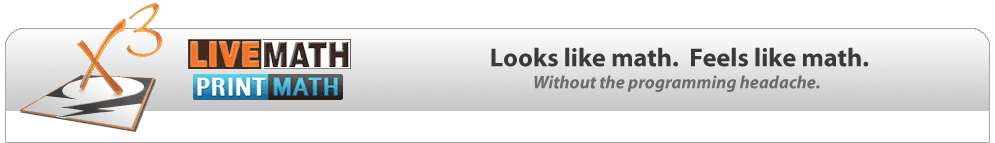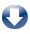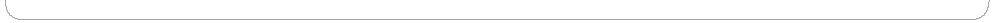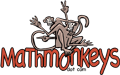Download 30-Day Free Trial & Free SoftwareLiveMath Channel Screen Recordings HowTos & NewsLiveMath Docs Books & Screencast Movies

## Are You a Distance Calculus Student?

Click on Assignment Quick Menu To See Your E-Textbook Separated into Assignments
Your Access Codes Will Not Work Here!

# Course Curricula à la LiveMath

We proudly present these course curriculum projects that utilize LiveMath. These e-texts feature LiveMath notebooks, screencast recordings discussing the topics in the LiveMath notebooks, which also serve as a tutorial on using LiveMath, and "by hand" videos as complimentary instruction for certain topics where hand calculations are important for mathematical literacy.

These course packages have served as the core curricula for the Distance Calculus project for the past 20+ years.

You may view a selection of topics for DEMO purposes. If you like what you see, then you may purchase full access to one (or all) of the e-textbooks to gain access to all of the LiveMath notebooks and help movies.

### Video Format Preference Setting

Use MP4     Use WebM     Use Flash

 The Primitives of Precalculus by Robert Curtis When Distance Calculus was launched back in 1997, the first courses were Calculus I and higher. As enrollment grew, it became clear that a number of students needed more Precalculus practice before jumping into the Calculus curriculum. Out of this necessity was born the comprehensive (and exhaustive!) The Primitives of Precalculus, bridging the gap between a varied high school math background and the core skill requirements of the Calculus I course. We begin with hand-calculations of solving basic equations from Algebra I level, all the way through conic sections, series, vectors, and polar equations - topics usually found in a precalculus textbook but never actually covered in class. The pedagogical approach is dual in nature: Hand-Calculations With hand-calculation videos, start the explanation of precalculus topics. If a student has already mastered such calculations, this part goes more quickly; LiveMath Calculations Moving towards LiveMath usage, the topics are more thoroughly investigated, with the added challenge of learning how to drive LiveMath to obtain calculations equivalent to the hand calculations, and then beyond what you may accomplish by hand. Not boring review as is usually the case in these courses, but a fresh and alive approach that allows students with weaker Precalculus skills to progress along with students whose skills are stronger, all of which are reinforced via the expanded LiveMath investigations. Using both hand-skills and LiveMath skills as the foundation, we then advance through the Precalculus curriculum eventually leaning towards LiveMath and advanced and unique topics that cannot be studied without a technological tool.Such unique and advanced topics include: Determining the polynomial formula for a mystery function with complex roots by vertically shifting the graph to create real roots in the shift, reading off those real roots visually, and then shifting back to obtain the factorization with complex roots and finding the mystery polynomial through this analysis. Discovering trigonometric identities through the usage of 3D graphical comparisons. Applying rotation of axes to analyze a tilted conic sectionCalculus&LiveMath I by Bill Davis, Hortio Porta, Jerry Uhl One of the original Calculus Reform projects is the Calculus&Mathematica series from The Ohio State University and The University of Illinois at Champaign-Urbana. Developed nearly 40 years ago, C&M remains one of the most ground-breaking and non-traditional calculus reform project, and still in active use in the NetMath program at UIUC. Calculus&LiveMath is an e-text ported (with some enhancments) from the original Mathematica to LiveMath format, with additional commentary screencast movies, and a few hand-based calculation movies for key topics. This e-text serves as the core curriculum for the Distance Calculus Engineering-based first year calculus curriculum. This first book in the C&LM series is entitled: Growth, concentrating on differential calculus. Non-standard topics include a concentration on Percentage Growth Rate, a topic not found in most textbooks. Limits - usually the cornerstone of Calculus I - are not to be found, which is a fundamental tenet of C&M, interested instead to study patterns of growth, families of functions, and physical applications.

 Calculus&LiveMath II by Bill Davis, Hortio Porta, Jerry Uhl Accumulation. That's what the integral really means. Too often the concept of the integral is tied up into the Riemann Sum construction, with students not really learning the more fundamental concept of accumulationM, whose theme is returned to again and again in the development of integration theory. The Fundamental Theorem of Calculus is explored via looking at integral functions - functions created using the integral object, and then looking at derivatives of the integral functions. Something you can only study via the usage of high powered technology like LiveMath (or Mathematica). In the middle of this curriculum we study Green's Theorem and the relationship between double integrals and path integrals - a topic usually reserved for vector calculus a year forward in the traditional curriculum. With a tool like LiveMath, what then becomes the student of antidifferentiation? There are some hand techniques for mathematical literacy, but then we move into using these technological tools, understanding what techniques they are applying to "crack the integral", and even use advanced techniques like complex integrals and Euler's formula to attack trigonometric integrals.Calculus&LiveMath III by Bill Davis, Hortio Porta, Jerry Uhl Approximation. Just like decimals may approximate any real number, polynomial functions may approximate any real (well-behaved, differentiable) function. The (often dreary) traditional approach to sequences and series is replaced by a lively and useful approached based upon splines to approximate general functions. This leads naturally to Taylor's Theorem, and finding the relationship between degrees of points of contact, derivatives, and shape of curves, all leading to the ability to integrate functions once you have a polynomial approximation, and then winding back to sequences and convergence, upending the traditional order of topics. This spline approach in the curriculum converts the last portion of first year calculus into a wonderful finale, quite different than the expected "p-test, this converges, this doesn't" bucket of confusion that most students experience in Calculus III.

 Vector Calculus&LiveMath by Bill Davis, Hortio Porta, Jerry Uhl The utilization of LiveMath comes alive in the multivariable calculus curriculum, bringing the power of easy-to-create 3D graphs of curves and surfaces in LiveMath to a fresh and innovative exploration of the topics of Vector Calculus. The theme of accumulation features prominently, as it did in C&LM II, this time with the accumulation of vector fields dot producted with differential vectors, sometimes tangential, sometimes orthogonal, providing a unique development of path integrals. The course progresses to the theorems of Green, Stokes, and Gauss, which are all just variations of the Fundament Theorem of Multivariable Calculus. Of all of the C&LM texts, this Vector Calculus&LiveMath is felt by many to be the best of the best.MathMonkeys, LLC
9 Grapevine Avenue, Suite 4
Lexington, Massachusetts 02421 USA
Need Help With LiveMath?